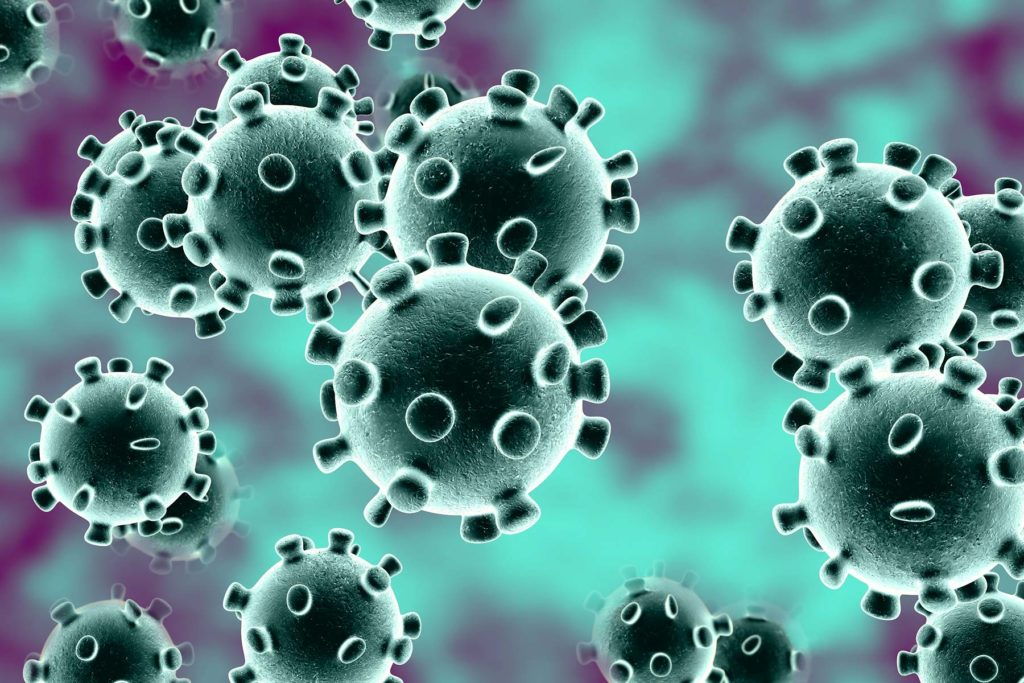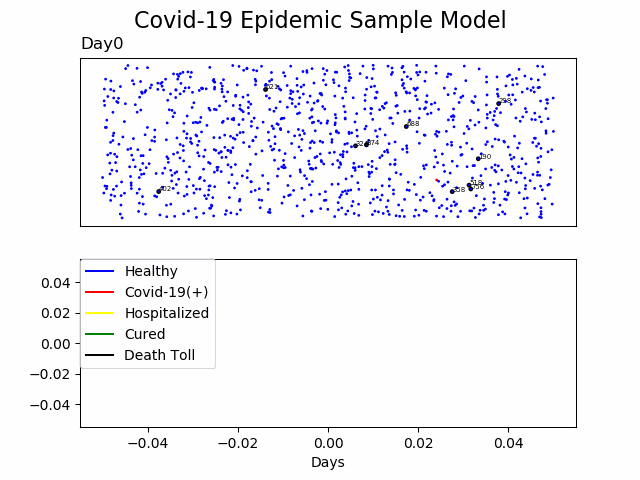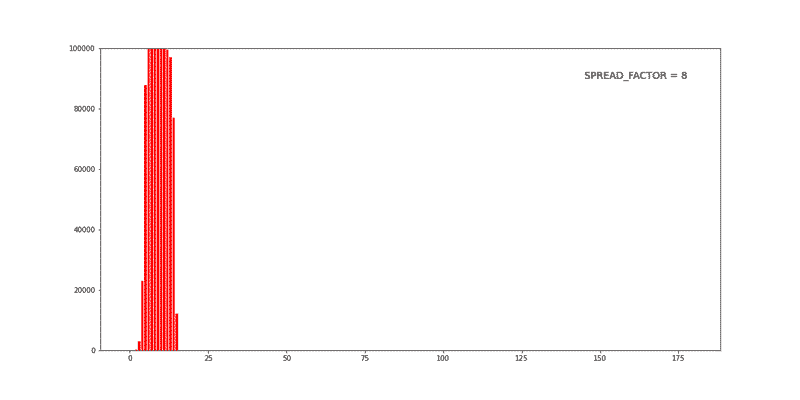# Get Started COVID-19How’s the craic?

## Get Started COVID-19

You are probably aware about Coronavirus or Covid-19 pandemic.

It is a good chance that you are reading this while self quarantined at your home, social distancing from public and like many, have very much extra time to deal with.

TL;DR
I came with the idea to create this blog post to maybe help or give some ideas for you to start something. if you are familiar with programming, Developer, Coder, Data something, you should give a go and try or start something.
This post is a collection of links, videos, tutorials and blogs that I found mixed with my opinion.

1. Some articles to start
2. Datasets
3. APIs
4. Dashboards
5. Ideas
6. Code

## 2.Datasets

In the wake of the Coronavirus outbreak, many data sources have been made available to the public in an effort to encourage research in the field. Recently, the White House and a group of leading researchers published the COVID-19 Open Research Dataset (CORD-19), which is available on Kaggle.
Kaggle calls data scientists to action on COVID-19

This link is the most famous or quoted for data source;
https://github.com/CSSEGISandData/COVID-19

## 3.APIs

But I found some APIs as well;

## 4.Dashboards

List of Dashboards;

Maybe the famous one;
https://coronavirus.jhu.edu/map.html

A complete one and interactive;
http://gabgoh.github.io/COVID/index.html

https://www.washingtonpost.com/graphics/2020/world/corona-simulator/

https://towardsdatascience.com/covid-19-open-source-dashboard-fa1d2b4cd985

https://towardsdatascience.com/covid-19-dashboard-b7f8b7c59431

https://www.covidvisualizer.com/

## 5.Ideas

There are several ideas around, and because the ideas are based on data analyses and similar topics Oracle OCI data Science is a good place to give a go.
Start free
Oracle DataScience Docs
video

You can use an open-source OCI Marketplace image as well, (GPU as optional), and talking about GPU, NVidia provides a free 90-day license to Parabricks

I created a list of links with some ideas that I found;

Just a simple start point to read the data and start playing with Python and Pandas
https://towardsdatascience.com/how-to-get-started-analyzing-covid-19-data-808822437c32

https://towardsdatascience.com/exploring-covid-19-research-publications-407f8c2aa842

https://towardsdatascience.com/machine-learning-the-coronavirus-9cb8352e1b36

https://medium.com/@noahhaber/flatten-the-curve-of-armchair-epidemiology-9aa8cf92d652

https://theconversation.com/how-to-model-a-pandemic-134187

There are some initiatives in the maker community as well;

https://www.forbes.com/sites/alexandrasternlicht/2020/03/18/theres-a-shortage-of-ventilators-for-coronavirus-patients-so-this-international-group-invented-an-open-source-alternative-thats-being-tested-next-week/#58ddd9493ba0

ventilator hackathon

Ultimate Medical Hackathon: How Fast Can We Design and Deploy an Open Source Ventilator?

Facebook group – Open Source COVID19 Medical Supplies

And some ideas regarding image analysis

https://www.pyimagesearch.com/2020/03/16/detecting-covid-19-in-x-ray-images-with-keras-tensorflow-and-deep-learning/

https://towardsdatascience.com/detecting-covid-19-induced-pneumonia-from-chest-x-rays-with-transfer-learning-an-implementation-311484e6afc1

https://github.com/ieee8023/covid-chestxray-dataset

There are some Hackathons around as well;

https://covid-global-hackathon.devpost.com

https://www.codevscovid19.org/

You can search #BuildforCOVID19

## 6.Code

1) You can build your own epidemic model at home.

I just made some small changes

 123456789101112131415161718192021222324252627282930313233343536373839404142434445464748495051525354555657585960616263646566676869707172737475767778798081828384858687888990919293949596979899100101102103104105106107108109110111112113114115116117118119120121122123124125126127128129130131132133134135136137138139140141142143144145146147148149150151152153154155156157158159160161162163164165166167168169170171172173174175176177178179180181182183184185186187188189190191192193194195196197198199200201202203204205206207208209210211212213214215216217218219220221222223224225226227228229230231232233234235236237238239240241242243244245246247248249250251252253254255256257258259260261262263264265266267268 import pandas as pd import matplotlib.pyplot as plt import math import glob import random from PIL import Image def point(xlimit,ylimit):     x = random.uniform(0,xlimit)     y = random.uniform(0,ylimit)     return x,y def Generate(GrupSize,xlimit,ylimit):     df = pd.DataFrame(columns='X,Y,Covid-19,Day'.split(','))         for i in range(GrupSize):         df.loc[i,'X'], df.loc[i,'Y'] = point(xlimit,ylimit)         df.loc[i,'Covid-19'] = False         samplesize = math.floor(GrupSize/100)     MoversList = df.sample(n = samplesize).index.values.tolist()         StatofDay = pd.DataFrame(columns='Healthy,Covid-19(+),Hospitalized,Cured,Dead'.split(','))     return df, StatofDay, MoversList     def plt1color(df):     cols=[]     for l in df.index:         if df.loc[l,'Covid-19']==True: #Infected             cols.append('red')         elif df.loc[l,'Covid-19']==666: #Dead             cols.append('black')         elif df.loc[l,'Covid-19']==115: #Hospitalized             cols.append('yellow')         elif df.loc[l,'Covid-19']==7: #Cured             cols.append('green')         else:             cols.append('blue') #Healthy     return cols def plt2color(Stat):     cols=[]     for i in Stat.columns:         if i=='Covid-19(+)': #Infected             cols.append('red')         elif i=='Dead': #Dead             cols.append('black')         elif i=='Hospitalized': #Hospitalized             cols.append('yellow')         elif i=='Cured': #Cured             cols.append('green')         else:             cols.append('blue') #Healthy     return cols def Plot():     global df, fig, Stat, Day, Moverslist     cols=plt1color(df)     ld = ['Healthy','Covid-19(+)','Hospitalized','Cured','Death Toll']     axs.cla()     axs.scatter(df['X'],df['Y'],s=1,c=cols)     for i in MoversList:         axs.scatter(df.loc[i,'X'],df.loc[i,'Y'],s=6,facecolors='none', edgecolors='black')         axs.text(df.loc[i,'X']+0.02, df.loc[i,'Y']+0.02, str(i), fontsize=5)     cols=plt2color(Stat)     sDay = str(Day)     title = 'Day' + sDay     axs.set_title(title,loc='left')     axs.set_yticklabels([])     axs.set_xticklabels([])     axs.tick_params( #    axis='both',       # changes apply to the x-axis     which='both',      # both major and minor ticks are affected     bottom=False,      # ticks along the bottom edge are off     top=False,         # ticks along the top edge are off     right=False,      # ticks along the right edge are off     left=False,         # ticks along the left edge are off     labelbottom=False) # labels along the bottom edge are off     axs.cla()     axs.plot(Stat.Healthy,label=ld,color=cols)     axs.plot(Stat['Covid-19(+)'],label=ld,color=cols)     axs.plot(Stat.Hospitalized,label=ld,color=cols)     axs.plot(Stat.Cured,label=ld,color=cols)     axs.plot(Stat.Dead,label=ld,color=cols) #    axs.set_prop_cycle(color=cols)     axs.legend(bbox_to_anchor=(0, 1), loc='upper left', borderaxespad=0.)     plt.xlabel('Days') #    plt.show()     if Day<10 : sDay = '0' + sDay     title = 'Day' + sDay + '.png'     plt.savefig(title)     return def Png_to_gif():       # Create frames     frames = []     imgs = sorted(glob.glob("*.png"))     for i in imgs:         new_frame = Image.open(i)         frames.append(new_frame)             # Save into GIF     frames.save('png_to_gif.gif', format='GIF',           append_images=frames[1:],           save_all=True,           duration=500, loop=0) def infect(Person):     global df,Day     if random.random()>0.25 and Day>3 : return     if df.loc[Person,'Covid-19']==False:         df.loc[Person,'Covid-19'], df.loc[Person,'Day'] = True, Day         def Move(xlimit,ylimit):     """     Move Movers Randomly     """     global df, MoversList     for i in MoversList:         if (df.loc[i,'Covid-19']==115) or (df.loc[i,'Covid-19']==666) :            MoversList.remove(i)         df.loc[i,'X'], df.loc[i,'Y'] = (df.loc[i,'X']+random.uniform(1,xlimit/3))%xlimit, (df.loc[i,'Y']+random.uniform(1,ylimit/3))%ylimit def check(i,j):     global df, YesterdayPatients, Distlimit     Dist = math.sqrt((df.loc[i,'X']-df.loc[j,'X'])**2+(df.loc[i,'Y']-df.loc[j,'Y'])**2)     flag = ((YesterdayPatients[i]==True) ^ (YesterdayPatients[j]==True)) and Distlen(df[df['Covid-19']==True]):         return     df.loc[df[df['Covid-19']==True].sample(n = samplesize).index.values.tolist(),'Covid-19']=666     return def hospitilize():     global df     samplesize = math.floor(len(df[df['Covid-19']==True])*0.03)     if samplesize>len(df[df['Covid-19']==True]):         return     df.loc[df[df['Covid-19']==True].sample(n = samplesize).index.values.tolist(),'Covid-19']=115     return def cure():     global df, Day     df.loc[(df['Day']0 and Day<100:     log_file = open("Log.txt","a+")     if (list(Stat.loc[Day])==list(Stat.loc[Day-1])):         countsames +=1         if countsames>2 : break     else :         countsames = 0         YesterdayPatients = list(df['Covid-19'])     Tomorrow()     Plot()     Count(Day)     write_log(31*'-')     write_log('Day:',Day)     write_log(8*'- - ')         write_log(Stat.loc[Day])     log_file.close() Png_to_gif() Stat.to_excel('Stat.xlsx') Stat.plot(title='Statistical Data Vs. Days Passed') plt.savefig('Stat')2) You can visualize the impact of social distancing

I just made some small changes

 1234567891011121314151617181920212223242526272829303132333435363738394041424344 import numpy as np import pandas as pd import matplotlib.pyplot as plt DAYS = 180 POPULATION = 100000 SPREAD_FACTOR = 0.05 DAYS_TO_RECOVER = 10 INITIALLY_AFFECTED = 4 city = pd.DataFrame(data={'id': np.arange(POPULATION), 'infected': False, 'recovery_day': None, 'recovered': False}) city = city.set_index('id') firstCases = city.sample(INITIALLY_AFFECTED, replace=False) city.loc[firstCases.index, 'infected'] = True city.loc[firstCases.index, 'recovery_day'] = DAYS_TO_RECOVER stat_active_cases = [INITIALLY_AFFECTED] stat_recovered =  for today in range(1, DAYS):     city.loc[city['recovery_day'] == today, 'recovered'] = True     city.loc[city['recovery_day'] == today, 'infected'] = False     spreadingPeople = city[ (city['infected'] == True)]     totalCasesToday = round(len(spreadingPeople) * SPREAD_FACTOR)     casesToday = city.sample(totalCasesToday, replace=True)     # Ignore already infected or recovered people     casesToday = casesToday[ (casesToday['infected'] == False) & (casesToday['recovered'] == False) ]     # Mark the new cases as infected     city.loc[casesToday.index, 'infected'] = True     city.loc[casesToday.index, 'recovery_day'] = today + DAYS_TO_RECOVER     stat_active_cases.append(len(city[city['infected'] == True]))     stat_recovered.append(len(city[city['recovered'] == True])) title = "Spread Factor " + str(SPREAD_FACTOR).replace('.', '') fig = plt.figure(figsize=(16, 8)) plt.bar(np.arange(DAYS), stat_active_cases, color="red") plt.text(145, 90000, title, fontsize=14) #plt.show() plt.savefig(title)3) You cam use this code in both ideas to generate the animated gif.

 1234567891011121314151617181920 import glob from PIL import Image def Png_to_gif():       # Create frames     frames = []     imgs = sorted(glob.glob("*.png"))     for i in imgs:         new_frame = Image.open(i)         frames.append(new_frame)             # Save into GIF     frames.save('result.gif', format='GIF',           append_images=frames[1:],           save_all=True,           duration=500, loop=0) # Main --- Png_to_gif()

4) And of course, I did something with Raspberry Pi as well;

A simple Hello World example using GFX-Hat

 123456789101112131415161718192021222324252627282930313233343536373839404142434445464748495051525354555657585960616263646566676869707172737475767778798081 import requests import time import signal from gfxhat import touch, lcd, backlight, fonts from PIL import Image, ImageFont, ImageDraw resp = requests.get('https://thevirustracker.com/free-api?global=stats') if resp.status_code != 200:     # This means something went wrong.     raise ApiError('GET /tasks/ {}'.format(resp.status_code)) dic = resp.json() total_cases = 'total cases {}'.format(dic['results']['total_cases']) total_recovered = 'total recovered {}'.format(dic['results']['total_recovered']) total_deaths = 'total deaths {}'.format(dic['results']['total_deaths']) new_cases = 'total new cases today {}'.format(dic['results']['total_new_cases_today']) led_states = [False for _ in range(6)] width, height = lcd.dimensions() image = Image.new('P', (width, height)) draw = ImageDraw.Draw(image) font = ImageFont.truetype(fonts.AmaticSCBold, 12) text1 = total_cases text2 = total_recovered text3 = total_deaths text4 = new_cases #text = "Hello World" w, h = font.getsize(text1) x = (width - w) // 2 y = (height - h) // 2 draw.text((x, y-25), text1, 1, font) draw.text((x, y-13), text2, 1, font) draw.text((x, y), text3, 1, font) draw.text((x, y+13), text4, 1, font) def handler(ch, event):     if event == 'press':         led_states[ch] = not led_states[ch]         touch.set_led(ch, led_states[ch])         if led_states[ch]:             backlight.set_pixel(ch, 0, 255, 255)         else:             backlight.set_pixel(ch, 0, 255, 0)         backlight.show() for x in range(6):     touch.set_led(x, 1)     time.sleep(0.1)     touch.set_led(x, 0) for x in range(6):     backlight.set_pixel(x, 0, 255, 0)     touch.on(x, handler) backlight.show() for x in range(128):     for y in range(64):         pixel = image.getpixel((x, y))         lcd.set_pixel(x, y, pixel) lcd.show() try:     signal.pause() except KeyboardInterrupt:     for x in range(6):         backlight.set_pixel(x, 0, 0, 0)         touch.set_led(x, 0)     backlight.show()     lcd.clear()     lcd.show()